### Home > AC > Chapter 14 > Lesson 14.2.1.1 > Problem2-12

2-12.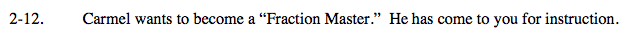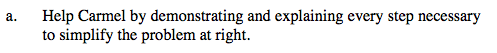Change to common denominator.

$\frac{8}{36}-\frac{9}{36}$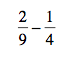Now just subtract the fractions.

$-\frac{1}{36}$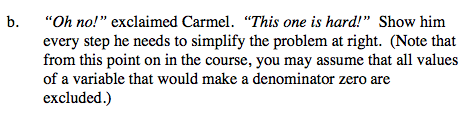Change to common denominator. (2xy)

$\frac{3\textit{y}}{2\textit{x}\textit{y}}+\frac{8}{2\textit{x}\textit{y}}$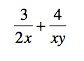Add the fractions to get one fraction.

$\frac{3\textit{y}+8}{2\textit{x}\textit{y}}$